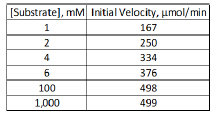Clutch Prep is now a part of Pearson
Ch. 6 - Enzymes and Enzyme KineticsWorksheetSee all chapters

# Kcat

See all sections
Sections
Enzymes
Enzyme-Substrate Complex
Lock and Key Vs. Induced Fit Models
Optimal Enzyme Conditions
Activation Energy
Types of Enzymes
Cofactor
Catalysis
Electrostatic and Metal Ion Catalysis
Covalent Catalysis
Reaction Rate
Enzyme Kinetics
Rate Constants and Rate Law
Reaction Orders
Rate Constant Units
Initial Velocity
Vmax Enzyme
Km Enzyme
Michaelis-Menten Assumptions
Michaelis-Menten Equation
Lineweaver-Burk Plot
Michaelis-Menten vs. Lineweaver-Burk Plots
Shifting Lineweaver-Burk Plots
Calculating Vmax
Calculating Km
Kcat
Specificity Constant

Concept #1: The Catalytic Constant Kcat

Concept #2: Calculating & Interpreting Kcat

Example #1: What is the turnover number for carbonic anhydrase if Vmax = 60,000 M/s and [E]T = 0.1 M?

Practice: To calculate the turnover number of an enzyme, you need to know:

Practice: If 10 μg of an enzyme (MW = 50,000 g/mol) is added to a solution containing a [substrate] 100 times greater than the Km, it catalyzes the conversion of 75 μmol of substrate into product in 3 min. What is the enzyme’s turnover #?

a) 1.25 x 105 min-1

b) 2.5 x 104 min-1

c) 1.5 x 102 min-1

d) 3.5 x 106 min-1

Concept #3: Kcat vs. Km

Practice: Studies with mutated forms of an enzyme show that changing some active-site amino acids decrease the enzyme’s turnover number (kcat) but do not affect the K m of the reaction. What is the best interpretation of these results?

Practice: The turnover number for an enzyme is known to be 5000 min -1. From the following set of data, determine both the Km and the total amount of enzyme ET.A) What is the Km of the enzyme?

a) 1 mM.

b) 2 mM.

c) 4 mM.

d) 1000 mM.

B) What is the total amount of enzyme?